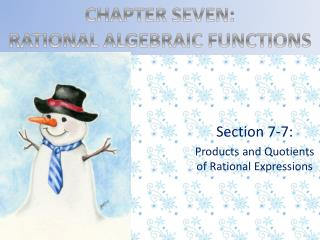# Section 7-7: Products and Quotients of Rational Expressions - PowerPoint PPT PresentationDownload PresentationSection 7-7: Products and Quotients of Rational Expressions

Download Presentation## Section 7-7: Products and Quotients of Rational Expressions

- - - - - - - - - - - - - - - - - - - - - - - - - - - E N D - - - - - - - - - - - - - - - - - - - - - - - - - - -
##### Presentation Transcript

1. CHAPTER SEVEN: RATIONAL ALGEBRAIC FUNCTIONS Section 7-7: Products and Quotients of Rational Expressions

2. Objectives: • Be able to multiply or divide several rational expressions and simplify the result.

3. Multiplication • To multiply two fractions, multiply their numerators together and multiply their denominators together. That is:

4. Example:

5. Division • Division of fractions is carried out by first applying the definition of division:

6. Example:

7. Simplified • A rational expression is simplified when its numerator and denominator have no common factors other than 1. • We simplify rational expressions by canceling.

8. Canceling • Canceling in a fraction means dividing the numerator and denominator of the fraction by the same common factor.

9. Example:

10. Example:

11. Complex Fractions

12. HOMEWORK: PAGE 360 #5-33 odds, and#49